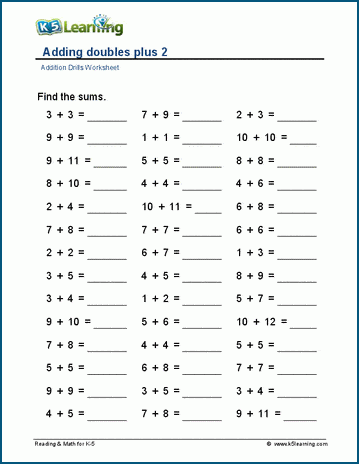A variety of addition worksheets aimed at gaining fluency in the basic addition facts up to 12 + 12.

We also have multi-digit addition worksheets and thousands of math worksheets by grade level.

Addition tables 1-10 (ordered)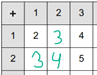## Addition tables - random order

Addition tables, 1-10, with hints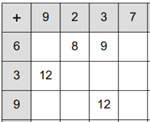Sums 5 or less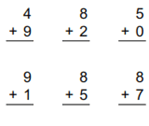Sums of 10 or less

Adding within 5 (horizontal)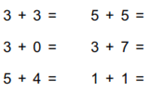Addition facts (horizontal - 80 questions)

Missing addends, within 0-5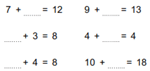Complements of 9 5 + __ = 9
Making 10  3 + __ = 10

Adding zeros to numbers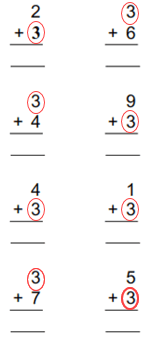## Circle drills

Circle drills (addition) 0-5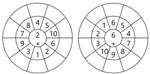## Number bonds

Number bonds of 5, 6 and 7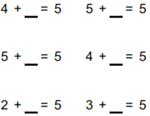Number bonds of 8, 9 and 10
Number bonds of 11-15
Number bonds of 16-20

Adding doubles 5 + 5 = __
Adding doubles plus 1 5 + 6 = __
Adding doubles plus 2 5 + 7 = __
Adding doubles minus 1 5 + 4 = __
Adding doubles minus 2 5 + 3 = __
Adding doubles and near doubles Mixed